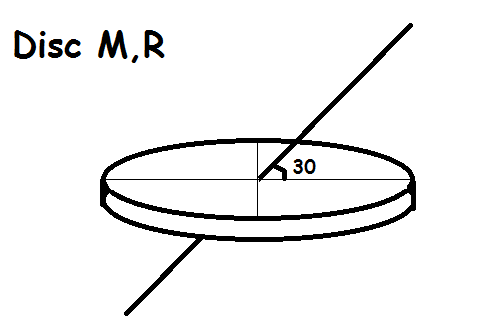# Moment of inertia

Find the moment of inertia of a solid disc of mass $M$ and radius $R$ about an axis which passes through the center and makes an angle of $30 ^\circ$ with the plane of disc as shown in figure.If the answer is of form $\dfrac{a}{b}MR^{2}$, find $a + b$. where a,b are coprime

×